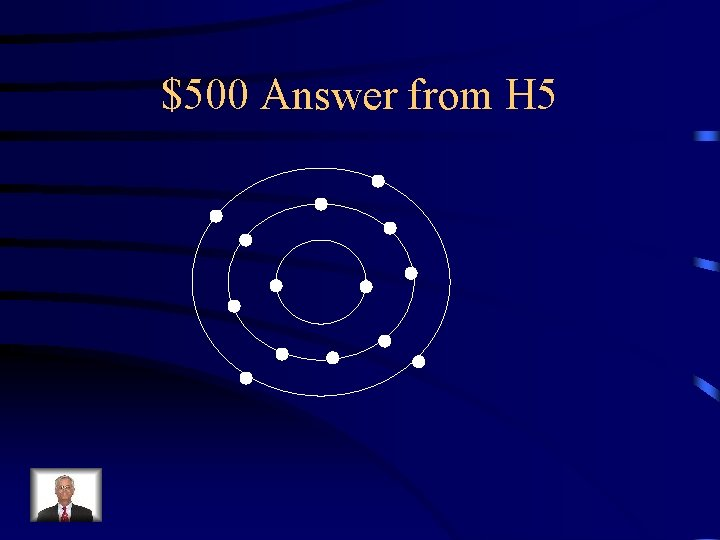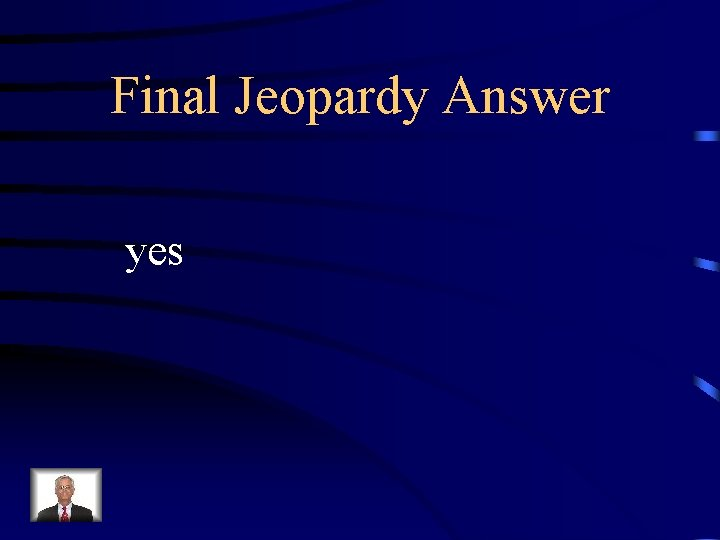# Jeopardy Atomic Models Atomic Particles Calculate Particles Isotopes

• Slides: 53Jeopardy Atomic Models Atomic Particles Calculate Particles Isotopes Valence Electrons Q \$100 Q \$100 Q \$200 Q \$200 Q \$300 Q \$300 Q \$400 Q \$400 Q \$500 Q \$500 Final Jeopardy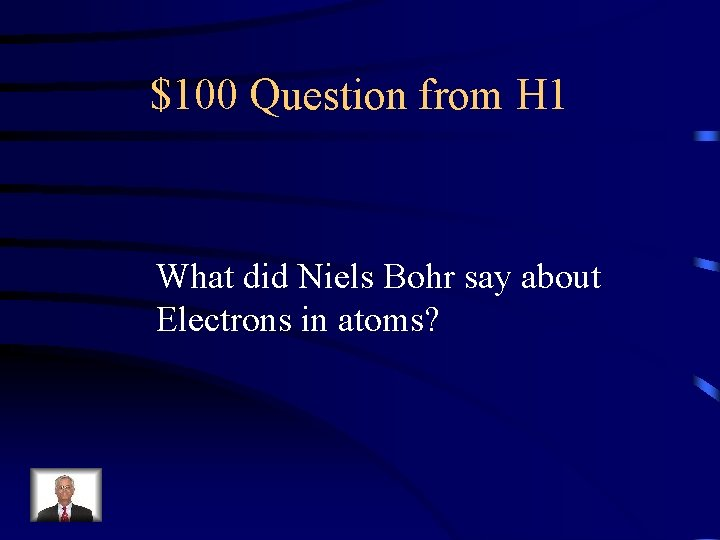\$100 Question from H 1 What did Niels Bohr say about Electrons in atoms?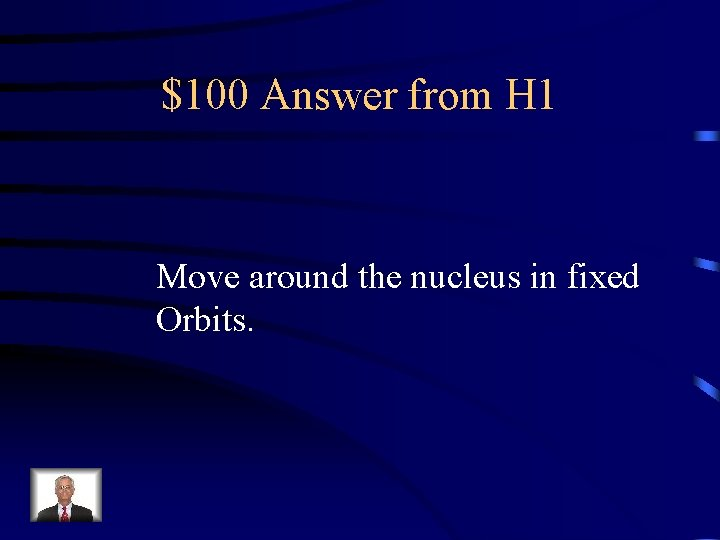\$100 Answer from H 1 Move around the nucleus in fixed Orbits.\$200 Question from H 1 What did J. J. Thomson discover Was part of an atom?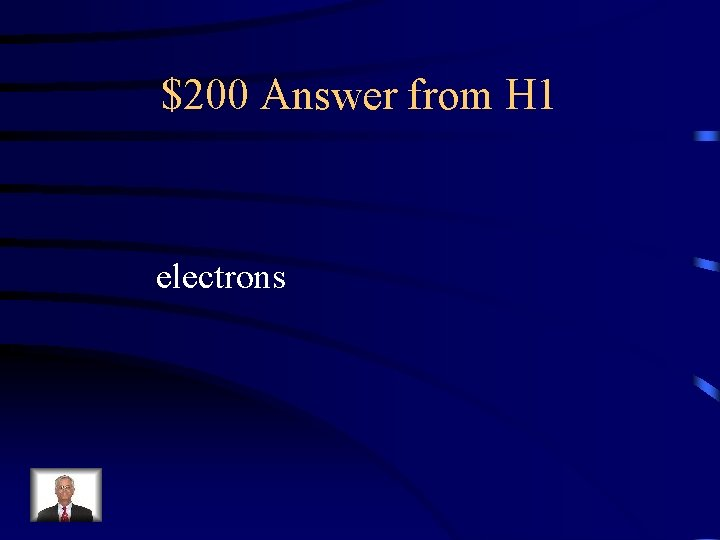\$200 Answer from H 1 electrons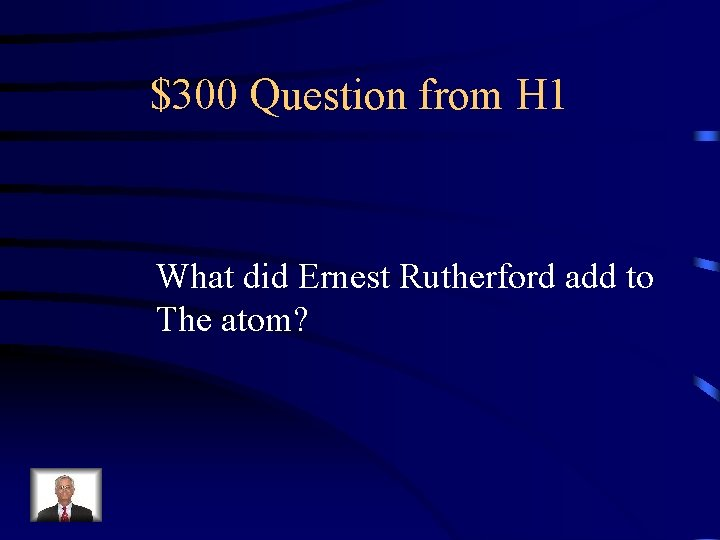\$300 Question from H 1 What did Ernest Rutherford add to The atom?\$300 Answer from H 1 nucleus\$400 Question from H 1 How did J. J. Thomson prove Electrons existed?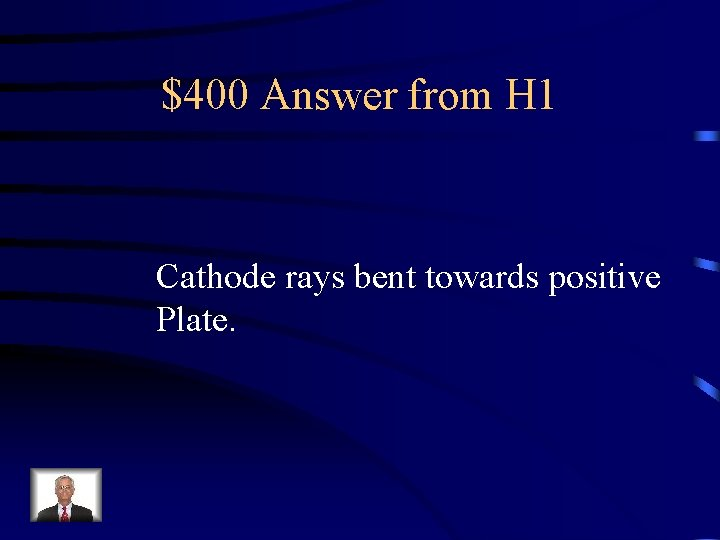\$400 Answer from H 1 Cathode rays bent towards positive Plate.\$500 Question from H 1 How did Ernest Rutherford prove That the nucleus existed?\$500 Answer from H 1 Fired positive particles at gold foil. Some particles were deflected.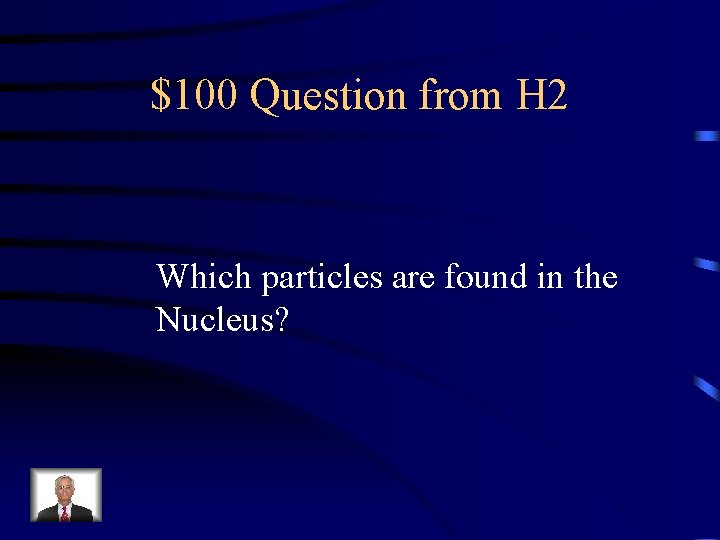\$100 Question from H 2 Which particles are found in the Nucleus?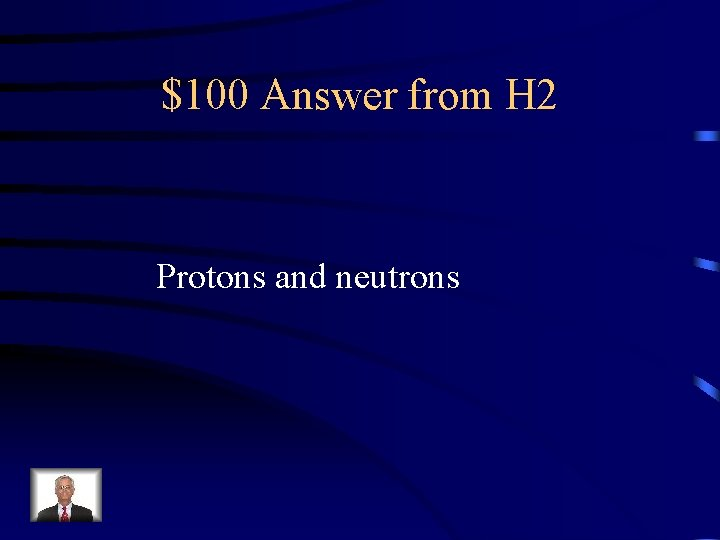\$100 Answer from H 2 Protons and neutrons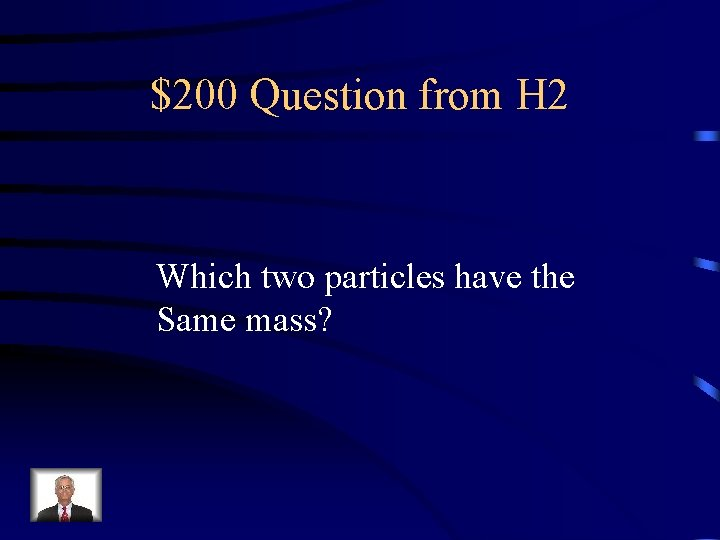\$200 Question from H 2 Which two particles have the Same mass?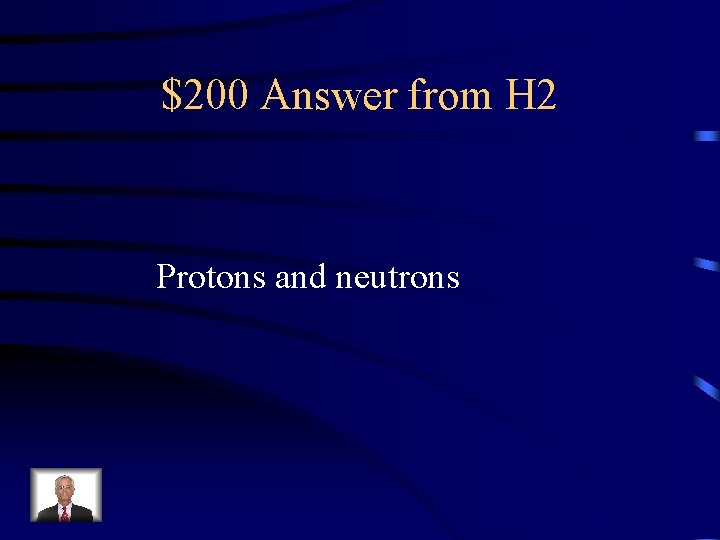\$200 Answer from H 2 Protons and neutrons\$300 Question from H 2 Which particle has a negative charge?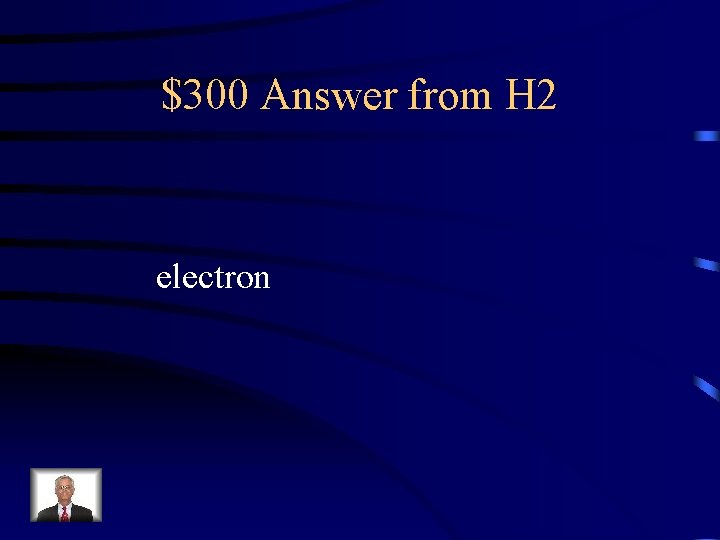\$300 Answer from H 2 electron\$400 Question from H 2 Which particle has no charge?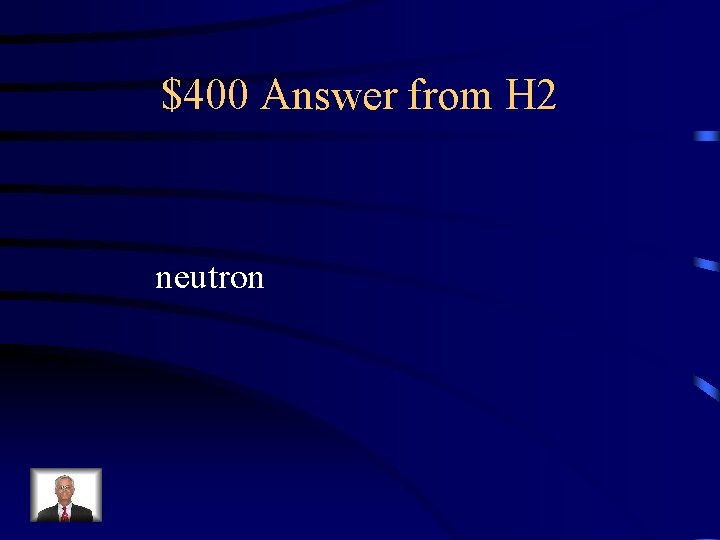\$400 Answer from H 2 neutron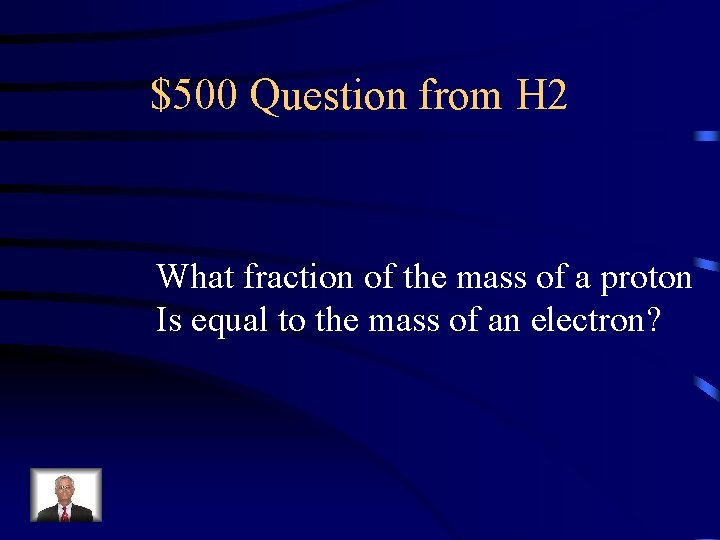\$500 Question from H 2 What fraction of the mass of a proton Is equal to the mass of an electron?\$500 Answer from H 2 1/1840\$100 Question from H 3 Lead has an atomic number of 82. How many protons does a lead Atom have?\$100 Answer from H 3 82\$200 Question from H 3 An atom of yttrium has a mass number Of 89 and has 50 neutrons. How many Protons does the atom have?\$200 Answer from H 3 39\$300 Question from H 3 How many electrons does an atom Of francium have? Atomic#=87 Mass#=223\$300 Answer from H 3 87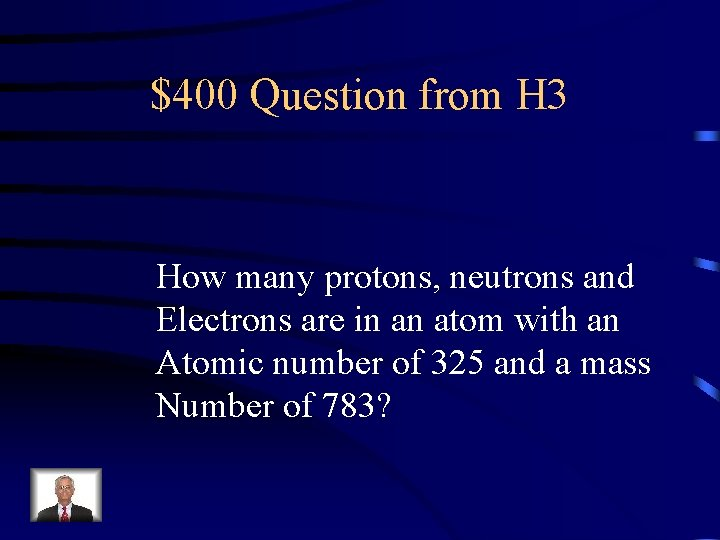\$400 Question from H 3 How many protons, neutrons and Electrons are in an atom with an Atomic number of 325 and a mass Number of 783?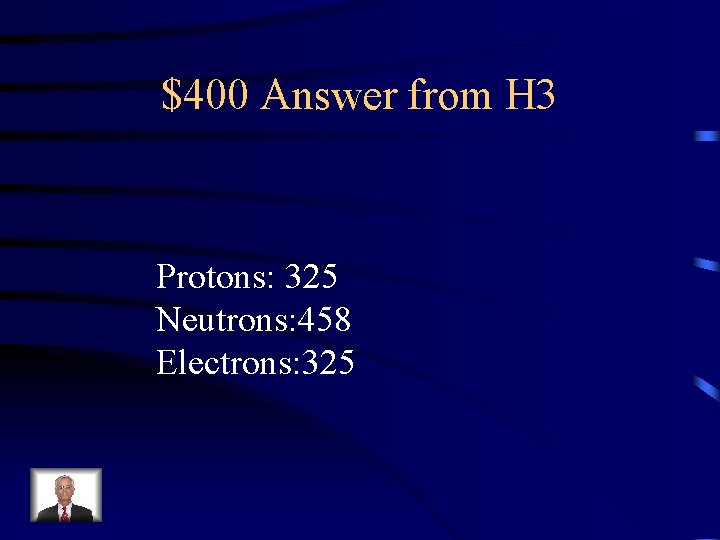\$400 Answer from H 3 Protons: 325 Neutrons: 458 Electrons: 325\$500 Question from H 3\$500 Answer from H 3 Protons: 573 Neutrons: 654 Electrons: 573\$100 Question from H 4 What are the two differences between Isotopes?\$100 Answer from H 4 Neutrons and mass numbers\$200 Question from H 4 What number will be the same for Isotopes?\$200 Answer from H 4 Atomic number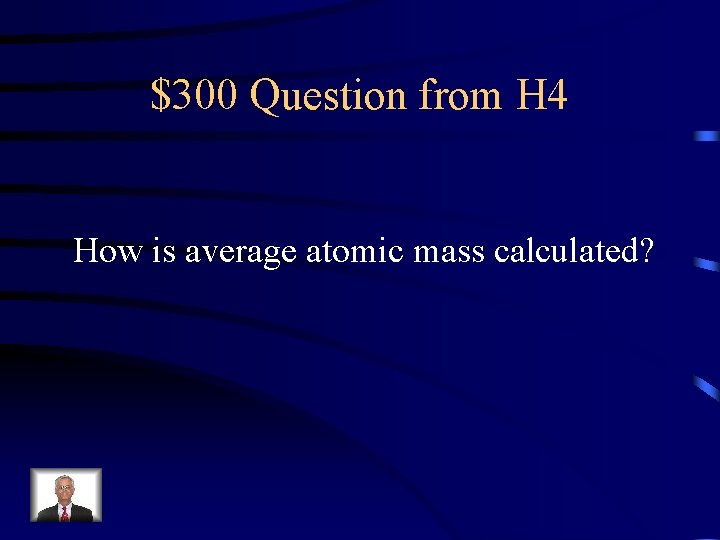\$300 Question from H 4 How is average atomic mass calculated?\$300 Answer from H 4 Weighted average of the mass of The isotopes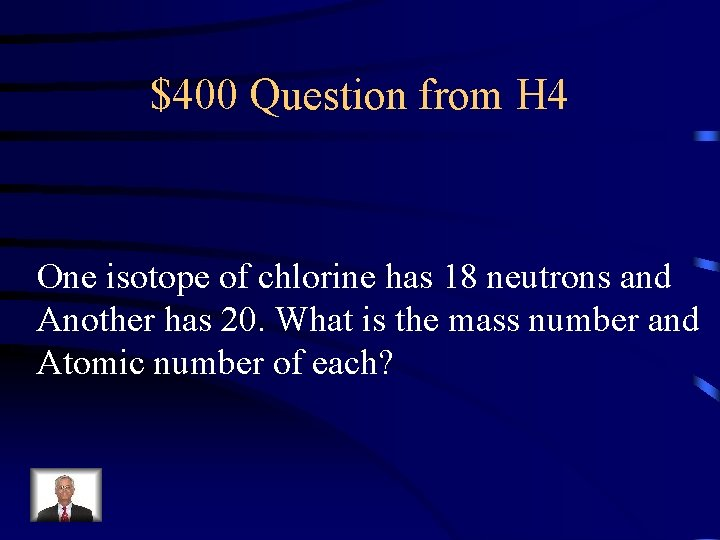\$400 Question from H 4 One isotope of chlorine has 18 neutrons and Another has 20. What is the mass number and Atomic number of each?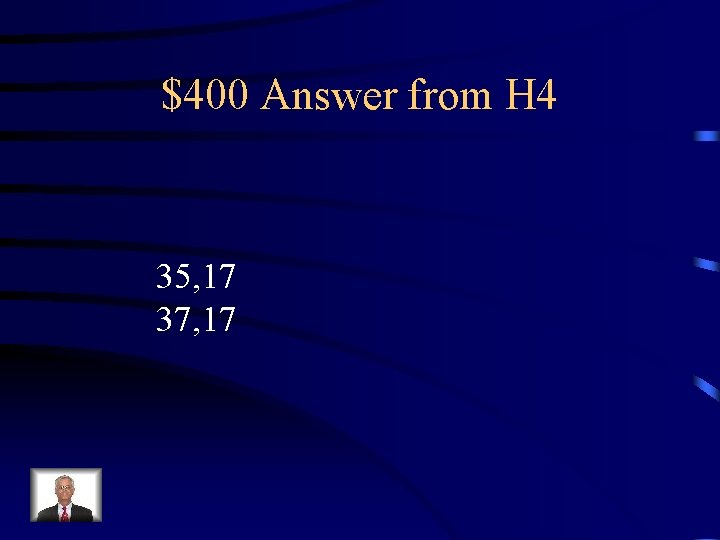\$400 Answer from H 4 35, 17 37, 17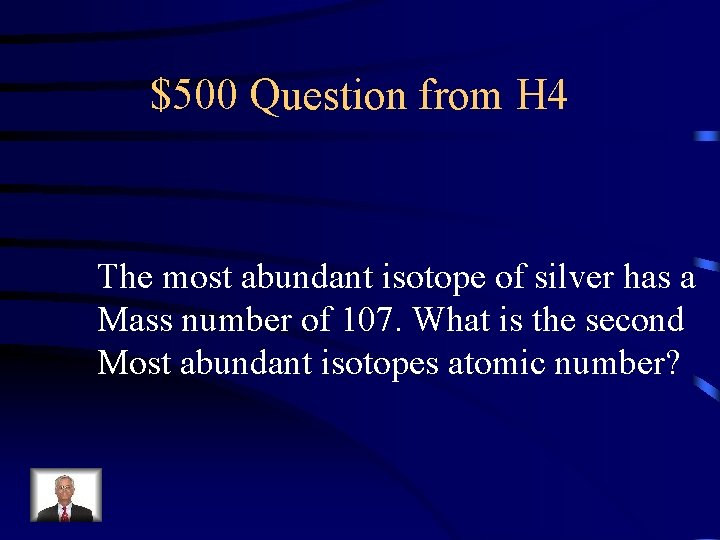\$500 Question from H 4 The most abundant isotope of silver has a Mass number of 107. What is the second Most abundant isotopes atomic number?\$500 Answer from H 4 47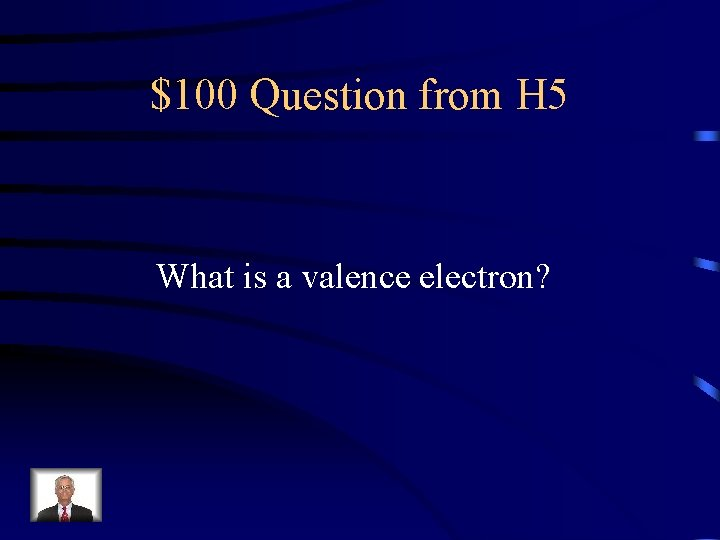\$100 Question from H 5 What is a valence electron?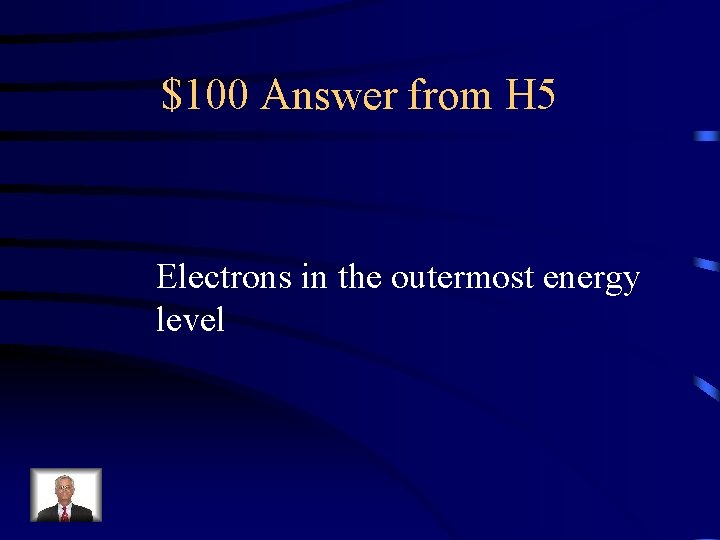\$100 Answer from H 5 Electrons in the outermost energy level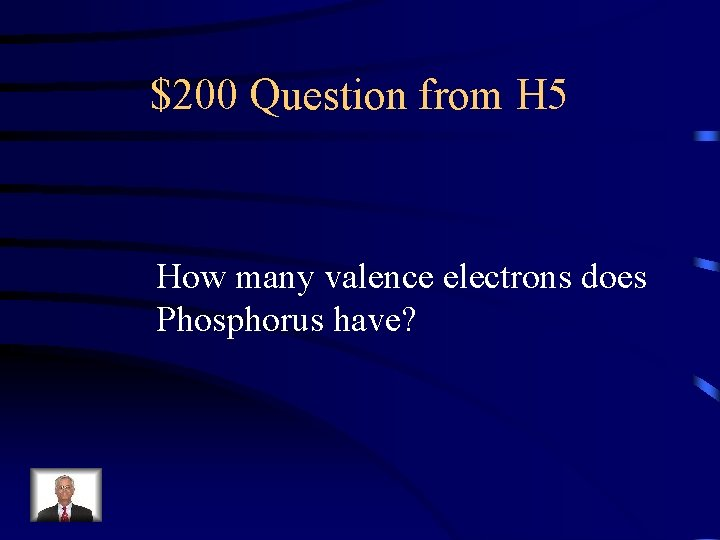\$200 Question from H 5 How many valence electrons does Phosphorus have?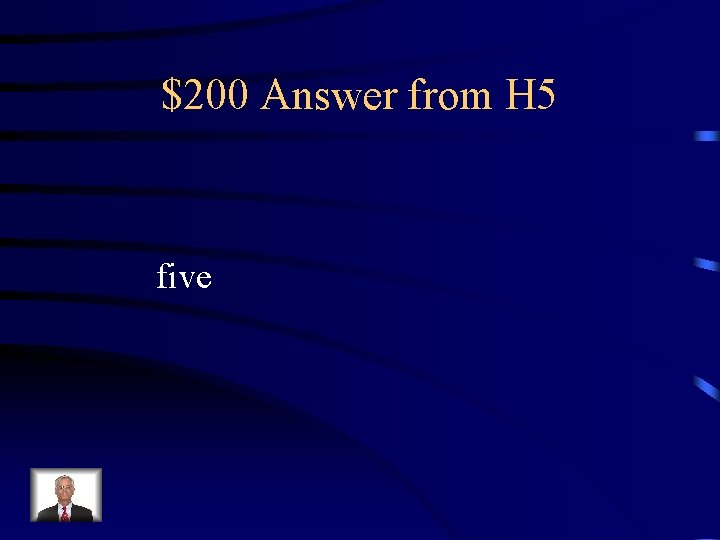\$200 Answer from H 5 five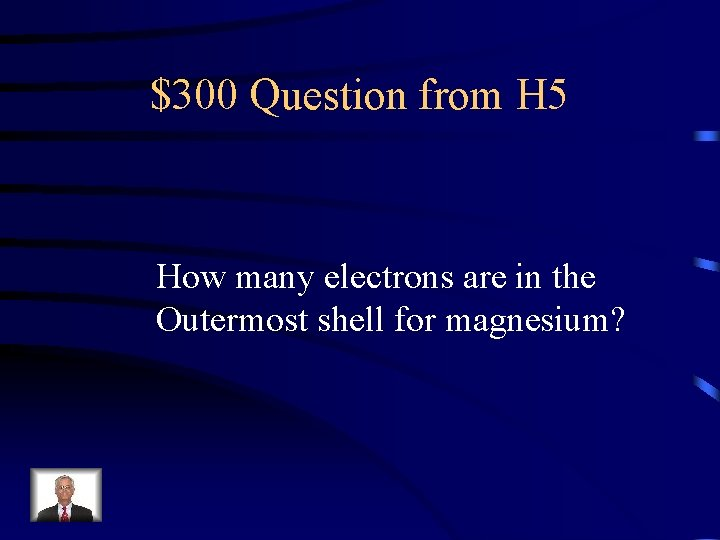\$300 Question from H 5 How many electrons are in the Outermost shell for magnesium?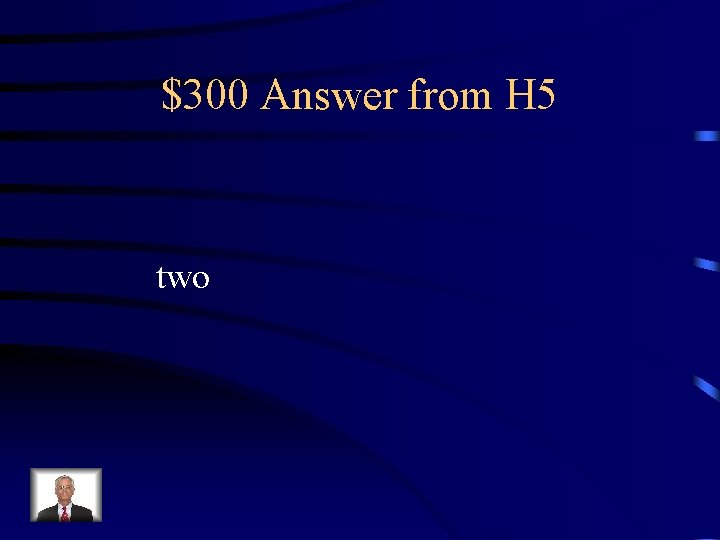\$300 Answer from H 5 two\$400 Question from H 5 Draw a Lewis Structure for selenium.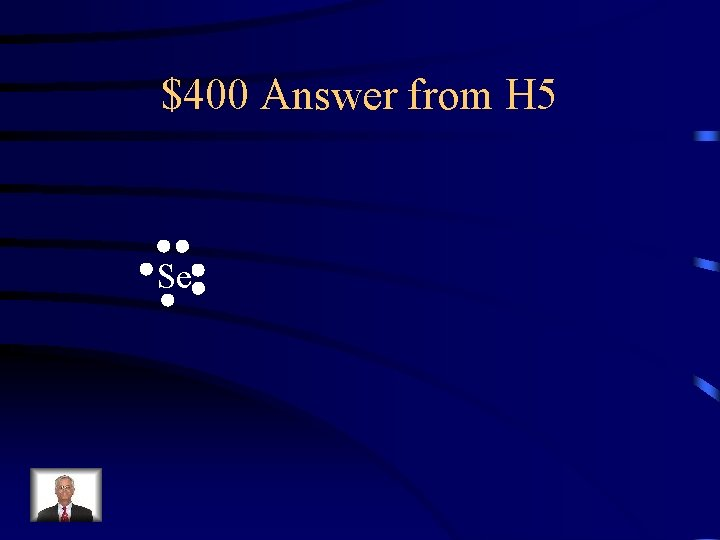\$400 Answer from H 5 Se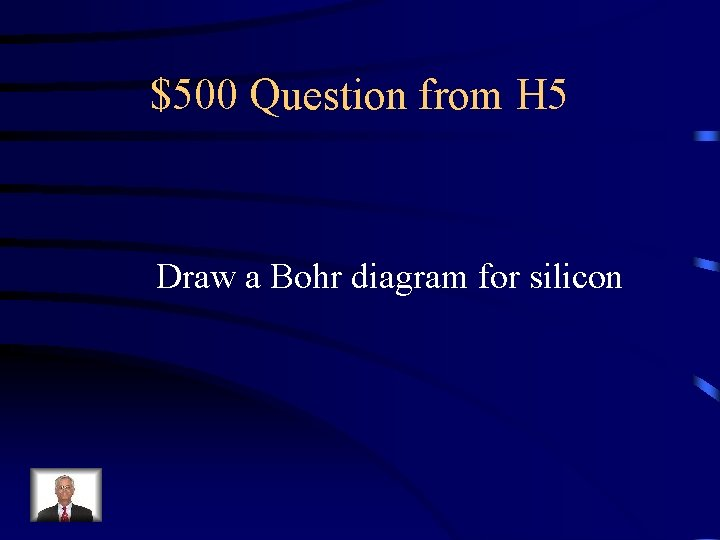\$500 Question from H 5 Draw a Bohr diagram for silicon